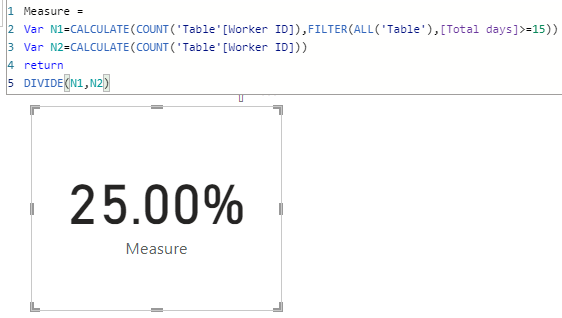cancel
Showing results for
Did you mean:Regular Visitor

## Calculate total workers where total days > than value as a %

Hi

I am trying to create a DAX to calculate how many workers have total days > than / buy total candidates

Data example below

for example, I need to count how many workers have total days worker > 15 / but total workers

 Wk Worker ID Days Worker 1 1 4 1 2 5 1 3 1 1 4 3 2 1 3 2 2 5 2 3 5 2 4 5 3 1 1 3 2 5 3 3 5 3 4 5

Thanks

3 ACCEPTED SOLUTIONSCommunity Champion

@Sully86 you mean like this (I changed to 13 casue you don't have any workers there with more then 15):

``````Measure =
COUNTROWS(
FILTER(
VALUES('Table'[Worker ID]),
"@Total Days", CALCULATE(SUM('Table'[Days Worker]))
),
[@Total Days] > 13
)
)``````Community Champion

@Sully86
my pleasure 🙂
Please don't forget to accept the previous message as a solution for community visabilty.
P.S. Check out my showcase report - got some high level stuff there. Sure you will find there a lot of cool ideas.
https://community.powerbi.com/t5/Data-Stories-Gallery/SpartaBI-Feat-Contoso-100K/td-p/2449543
Give it a thumbs up over there if you liked it 🙂Community Champion

@Sully86 you mean like this?

``````Measure =
COUNTROWS(
FILTER(
VALUES('Table'[Worker ID]),
"@Total Days", CALCULATE(SUM('Table'[Days Worker]))
),
[@Total Days] > 1 && [@Total Days] < 6
)
)``````7 REPLIES 7Community Support

Hi, @Sully86

``````Total days =
CALCULATE(SUM('Table'[Days Worker]),ALLEXCEPT('Table','Table'[Worker ID]))``````1. >= 15

``````Measure =
Var N1=CALCULATE(COUNT('Table'[Worker ID]),FILTER(ALL('Table'),[Total days]>=15))
Var N2=CALCULATE(COUNT('Table'[Worker ID]))
return
DIVIDE(N1,N2)``````2. >1 but < 6

``````Measure 1 =
Var N1=CALCULATE(COUNT('Table'[Worker ID]),FILTER(ALL('Table'),[Total days]>1&&[Total days]<6))
Var N2=CALCULATE(COUNT('Table'[Worker ID]))
return
DIVIDE(N1,N2)``````3. >6 but < 10

``````Measure 2 =
Var N1=CALCULATE(COUNT('Table'[Worker ID]),FILTER(ALL('Table'),[Total days]>6&&[Total days]<10))
Var N2=CALCULATE(COUNT('Table'[Worker ID]))
return
DIVIDE(N1,N2)``````Are these the outputs you expect?

Best Regards,

Community Support Team _Charlotte

If this post helps, then please consider Accept it as the solution to help the other members find it more quickly.Regular Visitor

@SpartaBI  how would I calc

>1 but < 6 days as one DAX

>6 but < 10 as another DAX

ThanksCommunity Champion

@Sully86 you mean like this?

``````Measure =
COUNTROWS(
FILTER(
VALUES('Table'[Worker ID]),
"@Total Days", CALCULATE(SUM('Table'[Days Worker]))
),
[@Total Days] > 1 && [@Total Days] < 6
)
)``````Regular Visitor

@SpartaBI awsome thank youRegular Visitor

@SpartaBI Thank you so muchCommunity Champion

@Sully86
my pleasure 🙂
Please don't forget to accept the previous message as a solution for community visabilty.
P.S. Check out my showcase report - got some high level stuff there. Sure you will find there a lot of cool ideas.
https://community.powerbi.com/t5/Data-Stories-Gallery/SpartaBI-Feat-Contoso-100K/td-p/2449543
Give it a thumbs up over there if you liked it 🙂Community Champion

@Sully86 you mean like this (I changed to 13 casue you don't have any workers there with more then 15):

``````Measure =
COUNTROWS(
FILTER(
VALUES('Table'[Worker ID]),
"@Total Days", CALCULATE(SUM('Table'[Days Worker]))
),
[@Total Days] > 13
)
)``````